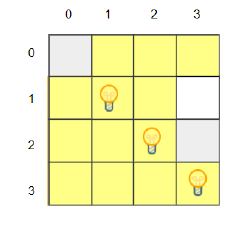# C++ Program to find out the number of illuminated cells in a grid

Suppose, we are given a grid of dimensions h * w. The cells in the grid can contain either bulbs or obstacles. A light bulb cell illuminates itself and the cells in its right, left, up, and down and the light can shine through the cells unless an obstacle cell blocks the light. An obstacle cell can not be illuminated and it blocks the light from a bulb cell from reaching the other cells. So, given the position of the bulb cells in the grid in array 'bulb' and the position of obstacle cells in the array 'obstacles', we have to find out the total number of cells in the grid that are illuminated.

So, if the input is like h = 4, w = 4, bulb = {{1, 1}, {2, 2}, {3, 3}}, obstacle = {{0, 0}, {2, 3}}, then the output will be 13.From the image, we can see the illuminated cells in the grid.

To solve this, we will follow these steps −

bulbSize := size of bulb
blockSize := size of obstacle
Define one 2D array grid
for initialize i := 0, when i < bulbSize, update (increase i by 1), do:
x := first value of bulb[i]
y := second value of bulb[i]
grid[x, y] := 1
for initialize i := 0, when i < blockSize, update (increase i by 1), do:
x := first value of obstacle[i]
y := first value of obstacle[i]
grid[x, y] := 2
result := h * w
Define one 2D array check
for initialize i := 0, when i < h, update (increase i by 1), do:
gd := 0
for initialize j := 0, when j < w, update (increase j by 1), do:
if grid[i, j] is same as 2, then:
gd := 0
if grid[i, j] is same as 1, then:
gd := 1
check[i, j] := check[i, j] OR gd
gd := 0
for initialize j := w - 1, when j >= 0, update (decrease j by 1), do:
if grid[i, j] is same as 2, then:
gd := 0
if grid[i, j] is same as 1, then:
gd := 1
check[i, j] := check[i, j] OR gd
for initialize j := 0, when j < w, update (increase j by 1), do:
k := 0
for initialize i := 0, when i < h, update (increase i by 1), do:
if grid[i, j] is same as 2, then:
k := 0
if grid[i, j] is same as 1, then:
k := 1
check[i, j] := check[i, j] OR k
k := 0
for initialize i := h - 1, when i >= 0, update (decrease i by 1), do:
if grid[i, j] is same as 2, then:
k := 0
if grid[i, j] is same as 1, then:
k := 1
check[i, j] := check[i, j] OR k
for initialize i := 0, when i < h, update (increase i by 1), do:
for initialize j := 0, when j < w, update (increase j by 1), do:
result := result - NOT(check[i, j])
return result

## Example

Let us see the following implementation to get better understanding −

#include <bits/stdc++.h>
using namespace std;

int solve(int h, int w, vector<pair<int, int>> bulb, vector<pair<int, int>> obstacle){
int bulbSize = bulb.size();
int blockSize = obstacle.size();
vector<vector<int>> grid(h, vector<int>(w, 0));
for (int i = 0; i < bulbSize; i++) {
int x = bulb[i].first;
int y = bulb[i].second;
grid[x][y] = 1;
}
for (int i = 0; i < blockSize; i++) {
int x = obstacle[i].first;
int y = obstacle[i].second;
grid[x][y] = 2;
}
int result = h * w;
vector<vector<bool>> check(h, vector<bool>(w, 0));
for (int i = 0; i < h; i++) {
bool gd = 0;
for (int j = 0; j < w; j++) {
if (grid[i][j] == 2)
gd = 0;
if (grid[i][j] == 1)
gd = 1;
check[i][j] = check[i][j] | gd;
}
gd = 0;
for (int j = w - 1; j >= 0; j--) {
if (grid[i][j] == 2)
gd = 0;
if (grid[i][j] == 1)
gd = 1;
check[i][j] = check[i][j] | gd;
}
}
for (int j = 0; j < w; j++) {
bool k = 0;
for (int i = 0; i < h; i++) {
if (grid[i][j] == 2)
k = 0;
if (grid[i][j] == 1)
k = 1;
check[i][j] = check[i][j] | k;
}
k = 0;
for (int i = h - 1; i >= 0; i--) {
if (grid[i][j] == 2)
k = 0;
if (grid[i][j] == 1) k = 1;
check[i][j] = check[i][j] | k;
}
}
for (int i = 0; i < h; i++)
for (int j = 0; j < w; j++)
result -= !check[i][j];
return result;
}
int main() {
int h = 4, w = 4;
vector<pair<int, int>> bulb = {{1, 1}, {2, 2}, {3, 3}}, obstacle = {{0, 0}, {2, 3}};
cout<< solve(h, w, bulb, obstacle);
return 0;
}

## Input

4, 4, {{1, 1}, {2, 2}, {3, 3}}, {{0, 0}, {2, 3}}


## Output

13